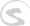Documents
Writing Docs Gigedit SFZ Instrument Scripts NKSP Language NKSP Reference

# real_to_int()

Performs a type cast from real number to integer number, which means it converts the passed `real-number` to an integer number and returns the latter as result value.

Any fractional value behind the decimal point of `real-number` is ignored by this conversion. So e.g. `real_to_int(3.999)` would return `3`. If that's not what you want, then you might consider using e.g. `round()` or `ceil()` before doing the actual type conversion with this function.

### Function Prototype

`real_to_int(real-number) `

### Arguments

Argument Name Data Type Description
`real-number` Real Number The real number to be converted.
[required]

### Return Value

Data Type Description
Integer Number Truncated Integer Number representation of the passed Real Number.

### Remarks

This functions accepts any standard unit and finalness for its argument `real-number`. The return value's standard unit and finalness is identical to the passed `real-number`'s one.

### Examples

None yet.

`int()`, `int_to_real()`, `round()`, `ceil()`